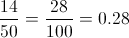## Example Questions

### Example Question #3 : Percentage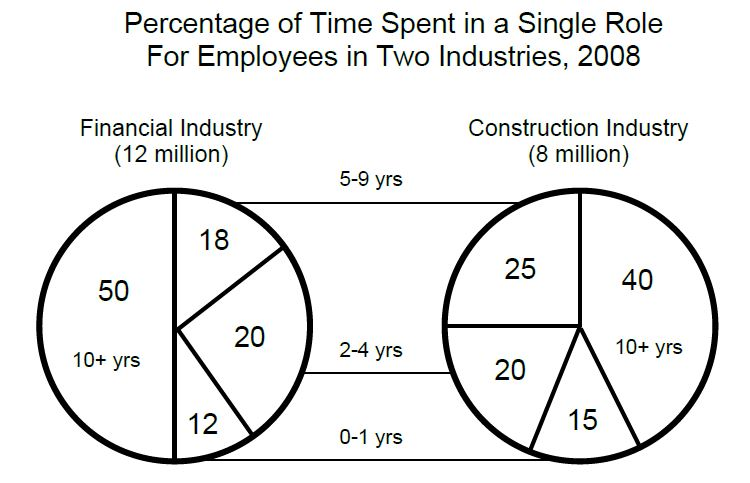If one of the employees across both industries were to be selected at random, what is the probability that the employee will be a construction industry worker who stayed in the same role for 5 years or more?

65%

20%

10%

17%

26%

26%

Explanation:

The first step is to figure out the percentage of construction employees that have stayed in the same role for 5 years or more—this would include both the "5 to 9 year" and "10+ years" ranges. This would be 0.25 + 0.4 = 65% of all construction employees.  To convert to the number of employees, we take the percentage of their total, 0.65 * 8,000,000 = 5,200,000 workers. However, since the probability we are attempting to find is of workers between both industries, we must add the 8 million to the 12 million = 20 million workers total. 5,200,000/20,000,000 = 0.26, or a 26% chance.

### Example Question #1 : Percentage

For every 1000 cookies baked, 34 are oatmeal raisin.

Quantity A: Percent of cookies baked that are oatmeal raisin

Quantity B: 3.4%

Quantity A is greater

The two quantities are equal.

Quantity B is greater

The relationship cannot be determined from the information given.

The two quantities are equal.

Explanation:

Simplify Quantity A by dividing the number of oatmeal raisin cookies by the total number of cookies to find the percentage of oatmeal raisin cookies. Since a percentage is defined as being out of 100, either multiply the resulting decimal by 100 or reduce the fraction until the denominator is 100. You will find that the two quantities are equal.

### Example Question #5 : Percentage

50 students took an exam. There were 4 A's, 9 B's, 15 C's, 8 D's, and the rest of the students failed. What percent of the students failed?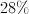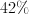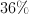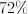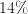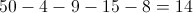students failed.